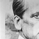1168 vues
Crossing 8 sma and 34 sma on the 1h chart (close) of EURUSD .
If sma (8) crossing up sma (34) then open a long on closed bar with +480 pips for the take profit and -950 pips for the stop loss.
If sma (8) crossing down sma (34) then open a short on closed bar with -480 pips for the take profit and +950 pips for the stop loss.
```//@version=2
strategy("8ma34 EURUSD 1h 480tp 950sl", shorttitle="MA", overlay=true, pyramiding=0, default_qty_value=10000, initial_capital=5000, currency="USD")
fastLength = input(8)
slowLength = input(34)
price = close

mafast = sma(price, fastLength)
maslow = sma(price, slowLength)

if (crossover(mafast, maslow))
strategy.entry("Long", strategy.long, comment=" ")

if (crossunder(mafast, maslow))
strategy.entry("Short", strategy.short, comment=" ")

strategy.exit("exit", "Long", profit = 480, loss = 950)
strategy.exit("exit", "Short", profit = 480, loss = 950)

```

## CommentairesHow it looks when sma (8) crossing sma (34).
On the chart showing prices of the entry, take and stop.
Répondre